# Natural numbers - 8th grade (13y) - math problems

#### Number of problems found: 164

• A three-digit numbers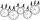Determine the total number of positive three-digit numbers that contain a digit 6.
• Drawing from a hatWhen drawing numbers from a hat from 1 to 35, we select random given numbers. What is the probability that the drawn numbers will be divisible by 8 and 2?
• MO 2016 Numerical axisCat's school use a special numerical axis. The distance between the numbers 1 and 2 is 1 cm, the distance between the numbers 2 and 3 is 3 cm, between the numbers 3 and 4 is 5 cm and so on, the distance between the next pair of natural numbers is always i
• Ratio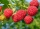Alena collected 7.8 kg of blueberries, 2.6 kg of blackberries, and 3.9 kg of cranberries. Express the ratio in the smallest natural numbers in this order.
• Two unknown numbersOne number is 2 less than a second number. Twice the second number is 14 less than 5 times the first. Find the two numbers.
• Self-counting machineThe self-counting machine works exactly like a calculator. The innkeeper wanted to add several three-digit natural numbers on his own. On the first attempt, he got the result in 2224. To check, he added these numbers again and he got 2198. Therefore, he a
• Seven-segmet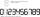Lenka is amused that he punched a calculator (seven-segment display) numbers and used only digits 2 to 9. Some numbers have the property that their image in the axial or central symmetry was again give some number. Determine the maximum number of three-di
• Real estate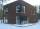Residential house has three entrances numbered by odd numbers arithmetic progression. The sum of the two numbers on the corner entrances is 50. Calculate the highest of this three numbers.
• Progression-12, 60, -300,1500 need next 2 numbers of pattern
• AlgebrogramSolve algebrogram for sum of three numbers: BEK KEMR SOMR ________ HERCI
• Balls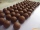From the bag with numbered balls (numbers 1,2,3,. ..20) we pick one ball. What is the probability of choosing a number containing 1?
• Coloured numbersMussel wrote four different natural numbers with coloured markers: red, blue, green and yellow. When the red number divides by blue, it gets the green number as an incomplete proportion, and yellow represents the remainder after this division. When it div
• Z7-I-4 stars 4949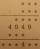Write instead of stars digits so the next write of product of the two numbers to be valid: ∗ ∗ ∗ · ∗ ∗ ∗ ∗ ∗ ∗ ∗ 4 9 4 9 ∗ ∗ ∗ ∗ ∗ ∗ 4 ∗ ∗
• Reminder and quotientThere are given numbers A = 135, B = 315. Find the smallest natural number R greater than 1 so that the proportions R:A, R:B are with the remainder 1.
• Positive integersSeveral positive integers are written on the paper. Michaella only remembered that each number was half the sum of all the other numbers. How many numbers could be written on paper?
• Unknown numberIf I reduced the sum of the numbers 70 and the unknown number three times, I would get 100. what is the unknown number?
• Rectangle diagonalsIt is given rectangle with area 24 cm2 a circumference 20 cm. The length of one side is 2 cm larger than length of second side. Calculate the length of the diagonal. Length and width are yet expressed in natural numbers.
• Divisors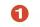Find all divisors of number 493. How many are them?
• Four-digit numbers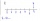Find four-digit numbers where all the digits are different. For numbers, the sum of the third and fourth digits is twice the sum of the first two digits, and the sum of the first and fourth digits is equal to the sum of the second and third digits. The di
• Probably memberLook at the series 2,6,25,96,285, ? What number should come next?

Do you have an interesting mathematical word problem that you can't solve it? Submit a math problem, and we can try to solve it.

We will send a solution to your e-mail address. Solved examples are also published here. Please enter the e-mail correctly and check whether you don't have a full mailbox.

Please do not submit problems from current active competitions such as Mathematical Olympiad, correspondence seminars etc...

Natural numbers - math word problems. Examples for 8th grade.